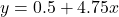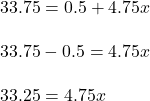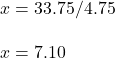## The water level in a pool is currently at 1/2 of an inch. Every hour, the water level increases 4.75 inches. How many hours will it take the

Question

The water level in a pool is currently at 1/2 of an inch. Every hour, the water level increases 4.75 inches. How many hours will it take the water level to reach 33.75 inches?

in progress 0
5 months 2021-08-18T11:58:30+00:00 1 Answers 1 views 0

## Answers ( )

7.1 hours

Step-by-step explanation:

Given data

Let the number of hours taken to fill the pool be x

the current quantity is 1/2 the capacity of the pool

we are given that the rate of flow = 4.75 in per hour

let the total amount of water after x hours be y, the expression for the quantity of water is given asif y= 33.75 in, let us find xdivide both sides by 4.75Hence, it will take 7.1 hours